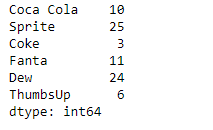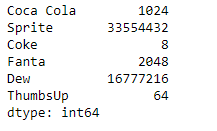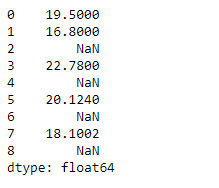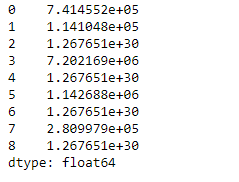# Python | Pandas Series.rpow()

Pandas series is a One-dimensional ndarray with axis labels. The labels need not be unique but must be a hashable type. The object supports both integer- and label-based indexing and provides a host of methods for performing operations involving the index.

Pandas` Series.rpow()` function return the exponential power of series and other, element-wise (binary operator rsub). It is equivalent to `other ** series`, but with support to substitute a fill_value for missing data in one of the inputs.

Syntax: Series.rpow(other, level=None, fill_value=None, axis=0)

Parameter :
other : Series or scalar value
fill_value : Fill existing missing (NaN) values
level : Broadcast across a level, matching Index values on the passed MultiIndex level

Returns : result : Series

Example #1: Use `Series.rpow()` function to raise a scalar value to the power of each element in the given Series object.

 `# importing pandas as pd ` `import` `pandas as pd ` ` `  `# Creating the Series ` `sr ``=` `pd.Series([``10``, ``25``, ``3``, ``11``, ``24``, ``6``]) ` ` `  `# Create the Index ` `index_ ``=` `[``'Coca Cola'``, ``'Sprite'``, ``'Coke'``, ``'Fanta'``, ``'Dew'``, ``'ThumbsUp'``] ` ` `  `# set the index ` `sr.index ``=` `index_ ` ` `  `# Print the series ` `print``(sr) `

Output :Now we will use `Series.rpow()` function to raise the scalar to the power of each element of the given series object.

 `# raise 2 to the power of each element in ` `# the sr object ` `selected_items ``=` `sr.rpow(other ``=` `2``) ` ` `  `# Print the returned Series object ` `print``(selected_items) `

Output :As we can see in the output, the `Series.rpow()` function has successfully returned a series object which is the result of exponentiation operation.

Example #2 : Use `Series.rpow()` function to raise a scalar value to the power of each element in the given Series object.

 `# importing pandas as pd ` `import` `pandas as pd ` ` `  `# Creating the Series ` `sr ``=` `pd.Series([``19.5``, ``16.8``, ``None``, ``22.78``, ``None``, ``20.124``, ``None``, ``18.1002``, ``None``]) ` ` `  `# Print the series ` `print``(sr) `

Output :Now we will use `Series.rpow()` function to raise the scalar to the power of each element of the given series object. We will substitute 100 at the place of all the missing values.

 `# raise 2 to the power of each element in ` `# the sr object ` `selected_items ``=` `sr.rpow(other ``=` `2``, fill_value ``=` `100``) ` ` `  `# Print the returned Series object ` `print``(selected_items) `

Output :As we can see in the output, the `Series.rpow()` function has successfully returned a series object which is the result of exponentiation operation.

My Personal Notes arrow_drop_upCheck out this Author's contributed articles.

If you like GeeksforGeeks and would like to contribute, you can also write an article using contribute.geeksforgeeks.org or mail your article to contribute@geeksforgeeks.org. See your article appearing on the GeeksforGeeks main page and help other Geeks.

Please Improve this article if you find anything incorrect by clicking on the "Improve Article" button below.

Article Tags :

Be the First to upvote.

Please write to us at contribute@geeksforgeeks.org to report any issue with the above content.